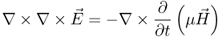# 2.5 Electromagnetic Waves

## 2.5.1 Wave Equation and Plane Waves

Now we will take a look at wave propagation in homogeneous, lossless,14 non-conducting media. Again we start with Maxwell's first and second equation in differential form

2.882.89We are interested in non-conducting media so the conductivity is zero (σ = 0) and there is no conduction current density. On the right-hand side of Ampere's law (Equation 2.88) only the displacement current densityremains. First we apply the curl operator and the constitutive relation(with μ = μ0μr) on Equation 2.89.

2.90Now we insert Equation 2.88 into Equation 2.90 by using the constitutive relation(with ε = ε0εr). Since we assume the medium to be time-invariant we can write the material parameters outside the derivative.

2.91

As in our discussion ...

Get RF and Microwave Engineering: Fundamentals of Wireless Communications now with the O’Reilly learning platform.

O’Reilly members experience live online training, plus books, videos, and digital content from nearly 200 publishers.